Home » Software » Change-Point Analyzer » Tutorial 2

## Change Point Analyzer Tutorial 2 - Multiple Observations Per Time Period

### Introduction

Before starting this tutorial you should have installed a copy of Change-Point Analyzer and have read Tutorial 1.  This tutorial will walk you through the steps of using this software to analyze a dataset containing multiple observations per time period.  We will be using the burst data contained in the file Burst.cpa.  This file was copied to your hard drive when Change-Point Analyzer was installed.

### Burst Data

Start Change-Point Analyzer.  Open the file Burst.cpa.  Your screen should appear as in Figure 1.  The Data window contains three tabs corresponding to three sealing stations.  Three samples were selected from each sealing station every half hour for 45 periods.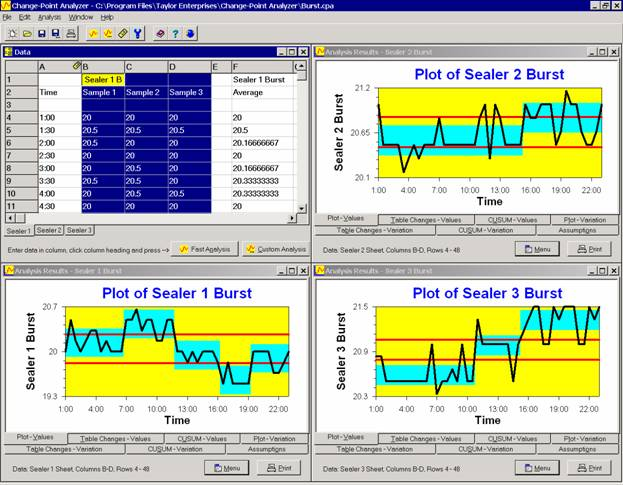Figure 1:  Burst Data

### Fast Analysis

Start by selecting the data to be analyzed.  The data of interest is contained in columns B-D.  To select these three columns, drag the mouse over their column headers.  The exact steps are:

1. Move the mouse cursor over the header for column B.
2. Press and hold down the left mouse button.
3. Move the mouse cursor across to the header for column D.
4. Release the left mouse button.

The three columns should now be highlighted as in Figure 1.

Column A has already been selected for labels as shown in Tutorial 1.  Perform a fast analysis by clicking on the Fast Analysis button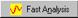at the bottom of the Data window.  As the analysis proceeds, the Analysis Status dialog box shown in Figure 2 is displayed.  The purpose of this dialog box is to assure you that the analysis is progressing.  Don’t worry about understanding the status messages.  Anything of importance will be displayed in the Analysis Results window once the analysis is completed.  Analyses take from less than a second to several minutes depending on the speed of your machine and the amount of data you are analyzing.  For the burst data, it should only take 2-5 seconds to complete the analysis.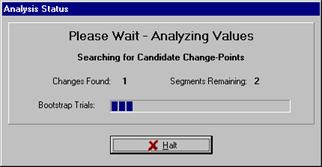Figure 2:  Analysis Status Dialog Box

Change-Point Analyzer automatically checks for outliers and a violation of assumptions.  If either is detected, a message appears describing the problem and recommending how to proceed.  A custom analysis is required.  Tutorial 4 describes the general procedure for performing custom analyses while Tutorials 5 and 6 describe how to handle outliers and a violation of assumptions.

### Interpreting the Results

Once the analysis has been completed, the results are shown in the Analysis Results window shown in Figure 3.  The results are displayed on 7 tabs.  The interpretation of these tabs is explained in Tutorial 1.  Only a few differences will be highlighted here.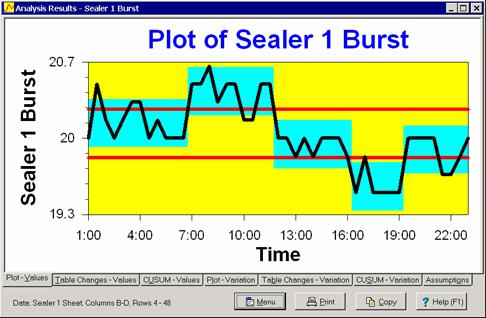Figure 3:  Analysis Results Window Showing Tab 1

When analyzing data with multiple observations per time period, Change-Point Analyzer calculates the average and standard deviation for each time period.  Tabs 1-3 show the results of performing a change-point analysis on these averages.  For example, Figure 3 shows Tab 1 contains a plot of the averages.  Tabs 4-6 show the results of performing a change-point analysis on the standard deviations.  Figure 4 shows Tab 4 contains a plot of the standard deviations.  The control limits on these two plots (red lines) represent an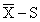control chart.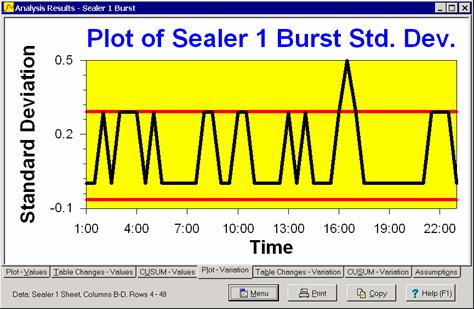Figure 4:  Analysis Results Window Showing Tab 4

With Change-Point Analyzer, handling multiple observations per time period is as simple as analyzing single observations per time period.

Scroll to Top